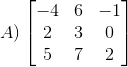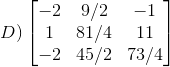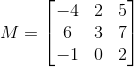top of page# If [A] and [B] are two matrices of same order, [A] + [B] is defined as matrix formed by sum of corresponding elements.# Square Matrix as a Sum of Symmetric and Skew-Symmetric Matrix:

Symm+skew symm# where,# (M + Trnas. of M) is a Symmetric matrix.# ​

GATE 2020: A matrix P is decomposed into its Symmetric part S and Skew-Symmetric part V. Find the matrix P.

Exmaples# ​

Matrix M = Symmetric part + Skew-Symmetric part

M = S + V

Adding S & V, M comes out to bebottom of page